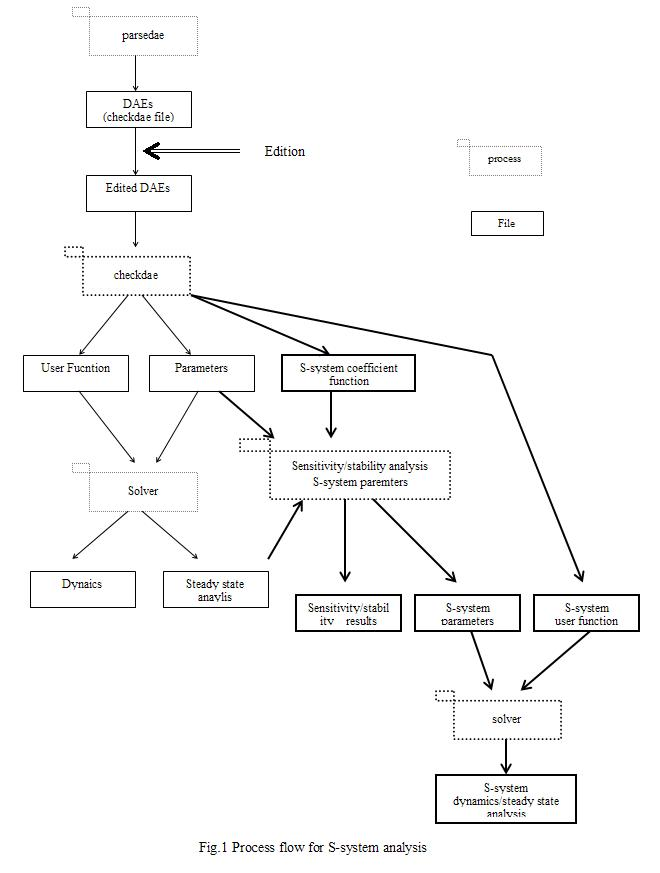# 1. Objectives

Sensitivity analysis shows how a biochemical system responds to perturbations or uncertainties. Among many methods for system analysis, the sensitivity and stability analysis is useful for characterizing the robustness of the mathematical at a steady-state level, and allows one to determine which parameters have the most effect on the model, or which factors cause to the system to oscillate. S-system is employed to analyze the various sensitivities and stability of the system at its steady state, because the use of S-system solves them in symbolic form. In this module, ordinary differential equations such as TT, CMA, GMA, and MM are converted into S-system to analyze the sensitivities and stability.

TT: ordinary transcription and translation equations

CMA : conventional mass action

GMA: general mass action

MM : simplified Michaelis-Menten equations

# 2. Introduction of S-system

We show how S-system is derived from ordinary differential equations, and how the stability and sensitivity are defined at the steady state.

## 2.1 S-system conversion

Ordinary differential equations are divided into the positive terms and negative terms: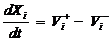,             (i = 1,....,n,....n+m)               (1),

where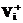is the sum of positive terms, and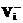is the sum of negative terms. Generally, S-system is given by: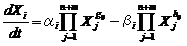(2),
where  X1,…, Xn are the dependent variables, whose values vary with time, and Xn+1,…,Xn+m are the independent variables, whose values are fixed as constants. When the concentrations of the dependent variables are given at the steady state, the coefficients (gij, hij, ai, bi) of S-system are solved from Eqs. (1, 2) in symbolic form: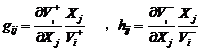(3)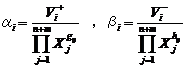(4)
where gij and hij are the kinetic order and
ai and bi are the rate constants. At the steady state: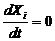,

we can take logarithms on both sides of Eq. (2), the equation reduces to a linear equation: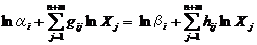,

or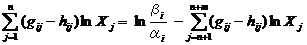(5).

Substituting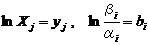. The equations (5) is changed to the vector-matrix expression: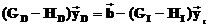(6).

We substitute the matrixes as follows,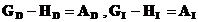to obtain the equation: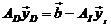(7)

where AD is the coefficient matrix of the kinetic order regarding dependent variables, AI is the coefficient matrix of the kinetic order regarding independent variables,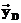is the solution vector of dependent variables,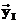is the vector of independent variables, and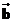is the vector regarding the rate constants. Consequently, the vector of the dependent variables is symbolically solved as: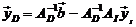(8).

## 2.2 Sensitivity analysis

We define five types of gains and sensitivities. The detailed derivation of them are described elsewhere. Here, we explain the mathematical definition of them.

### 2.2.1. Logarithmic Gain of a metabolite

In order to answer the question of how a relative change in an independent variable affect the steady-state concentration of a metabolite, the logarithmic gains of a metabolite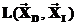is defined in terms of the coefficient matrixes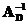and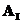as: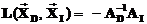(9).

### 2.2.2. Logarithmic gain of a flux

In order to answer the question of how a relative change in a flux affect the steady-state concentration of a metabolite, the logarithmic gains of a flux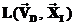is defined in terms of the coefficient matricesandas: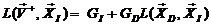(10).

### 2.2.3. Sensitivity with respect to a rate constant

In order to answer the question of how a relative change in a rate constant affect the steady state concentration of a metabolite, we solve the sensitivity with respect to rate constants, which is defined as: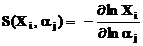(11),

and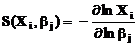(12).

These sensitivities are expressed using vectors and matrixes as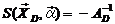(13),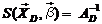(14).

### 2.2.4. Sensitivity of dependent variables with respect to a kinetic order

In order to answer the question of how a relative change in a kinetic order affect the steady-state concentration of a metabolite, we define the sensitivity with respect to the kinetic order as follows,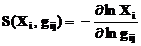(15),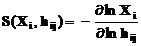(16).

The partial derivatives of Eq. (8) regarding gij are provided by: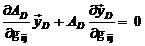,                                  (17)

or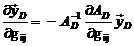.                                   (18)
Therefore,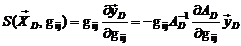(19)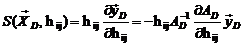(20).

### 2.2.5. Sensitivity of a flux to a rate constant

In order to answer the question of how a relative change in a rate constant affect the steady-state concentration of a flux, we define the sensitivity with respect to a rate constant.

We take logarithms in the first term of the right hand side of S-system: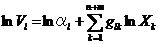(21)

Here, we differentiate to both side regarding lnαi to obtain: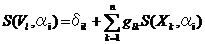(22)

In analogy, partial derivatives of Eq.(21) with respect to lnβi gives: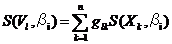(23).

### 2.2.6. Sensitivity of a flux with respect to a kinetic order

In order to answer the question of how a relative change in a kinetic order affect the steady-state concentration of a flux, we define the sensitivity with respect to a kinetic order.

The partial derivatives of Eq. (21) with respect of ln gij is provided as: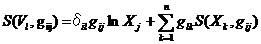(24).

In analogy, the partial derivatives of Eq. (21) with respect of ln hij is provided as: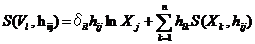(25).

## 2.3 Stability analysis

Defining the equation:

δXk = Xk XkS (XkS steady state),

we apply the first order approximation to Eq. (2) to obtain:(26),

or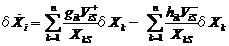(27),

As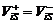, we define: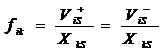to deduce to: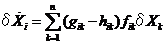(28).

Stability at the steady state is investigated by solving the eigenvalues of the coefficient matrix consisting of: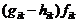.

# 3 Process flow

Fig. 1 shows the whole processes of the simulator, where the process flow of S-system mainly consists of three parts:

1) creation of the S-system coefficient file, which consists of the kinetic orders and rate constants that are solved in symbolic form at the steady state,

2) creation of the S-system parameter file (the coefficients and steady-state concentrations) necessary for sensitivity/stability analysis, which is made using the S-system coefficient function and the steady state concentrations.

3) simulation of S-system.

The checkdae module carries out the process of 1), following that the user function for ordinary differential equations (TT, CMA, GMA, MM) have been established. However, the checkdae module does not convert the differential and algebraic equations (DAEs), because they cannot be converted into S-system directly. The program module carries out the analysis of the sensitivities and stability of S-system by linking the S-system coefficient function to the steady-state concentrations. The concentrations at the steady state must be solved before this analysis.